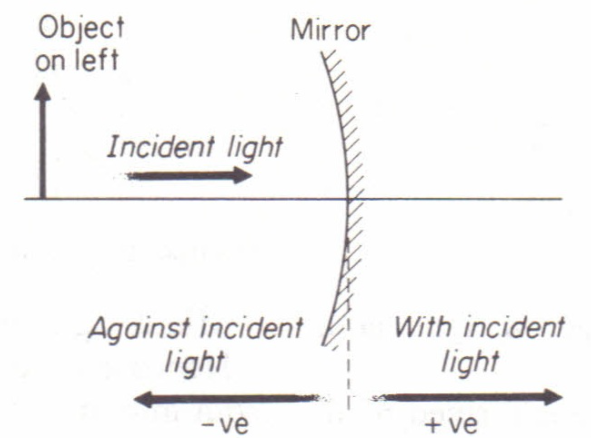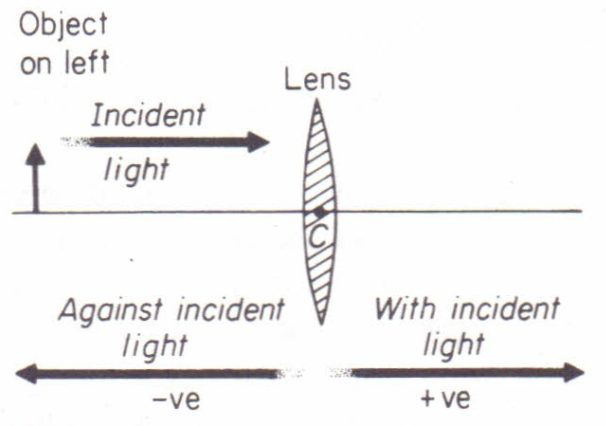# Explain the sign conventions of spherical mirror and spherical lens.

In optics, the main points we should remember about sign convention is:

• The direction of incident rays is always taken as a positive direction
• The pole or optical center is taken as origin(0). So distance should be measured from pole or optical centre

So, when an object is kept in front of a lens(or mirror), rays travel from the object to the lens(or mirror). These rays that travel from object to lens are called incident rays. The direction of incident rays is taken in a positive direction. The direction opposite this is taken as a negative direction.Concave mirror:

Since, object is always placed in front of the mirror, object distance is taken as negative.

Since, the centre of curvature and focus lie in front of the concave mirror, so radius of curvature and focal length is taken as negative in the case of concave mirror.

When an image is formed in front of the mirror, the distance of the image is taken as – (negative) and when the image is formed behind the mirror, the distance of the image is taken as + (positive).

Convex mirror:

Since an object is always placed in front of the mirror, the object distance is taken as negative.

Since the centre of curvature and focus lies behind the convex mirror, so radius of curvature and focal length are taken as + (positive) in the case of the convex mirror.

When image is formed in front of the mirror, the distance of image is taken as – (negative) and when image is formed behind the mirror, the distance of image is taken as + (positive).Lens:

Since the object is always placed in front of the lens, the object distance is taken as negative.

When image is formed in front of the lens, the distance of the image is taken as – (negative) and when image is formed behind the lens, the distance of image is taken as + (positive).

Updated on: 10-Oct-2022

122 Views